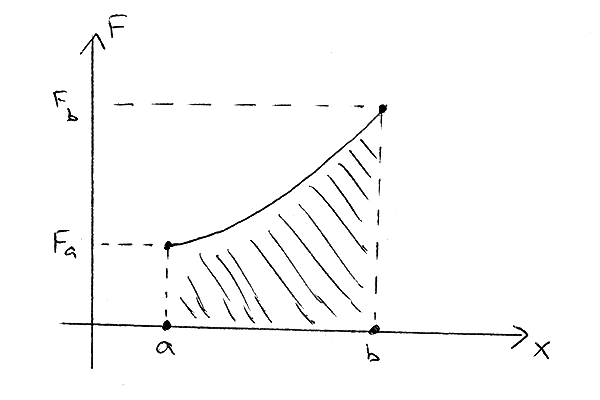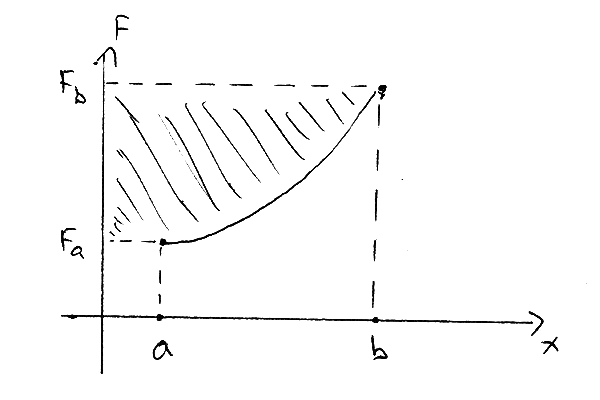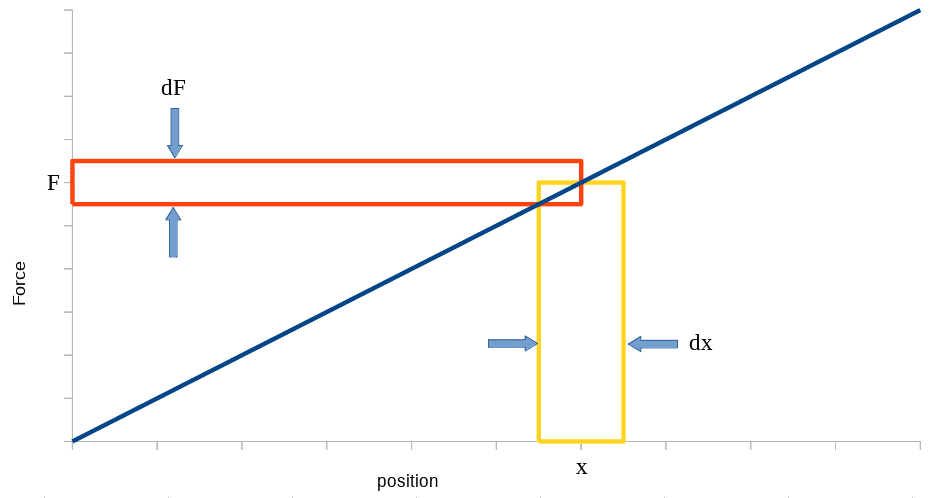# Why the work done is the area enclosed by the graph of F versus x on x Axis?

Why work done is area enclosed by graph of F v/s x on x Axis but not y axis.
Suppose we apply a force on object which is proportional to displacement as ##\vec f##=## \vec x##²then area enclosed by Force and displacement on x Axis is integral of ##\vec x##²but on y axis it should be integral of ##\vec x##.from this I conclude that something is not correct in my reasoning but I can't figure it out.

Work done by Force along the course C of the coordinate is
$$W=\int_C \mathbf{F}\cdot\mathbf{dl}=\int_C (F_xdx+F_ydy+F_zdz)$$
where ##\mathbf{dl}=(dx,dy,dz)## is tangent to C.
I do not find privileges among X-axis, Y-axis and Z-axis.

••vanhees71, Dale and etotheipi
Dale
Mentor
2020 Award
@Hemant your post is pretty unclear. Are you asking about x and y axes in terms of physical space or in terms of a graph?

@Hemant your post is pretty unclear. Are you asking about x and y axes in terms of physical space or in terms of a graph?
Graphs

etotheipi
Graphs

`If you have, as is fairly common, a graph of ##F_x## against ##x## (for instance, perhaps in the context of a spring), then the equation @mitochan gave reduces simply to ##W = \int_{x_{1}}^{x_{2}} F_x dx##. And this is the (signed) area bounded by the curve and the ##x## axis between the limits.

Dale
Mentor
2020 Award
Graphs

256bits
Gold Member
work done is area enclosed by graph of F v/s x on x
That's the definition.
and your post is a bit unclear.

Correct me if I have misinterpreted your post.
You are addressing, I believe something that is useful for linear (non-linear) systems that store energy, but not in any other cases, ie ones that don't store energy.
Co-energy is a useful concept in the analysis of certain systems, and you might not even know you are using it.
An explanation
http://www.encyclopedia-magnetica.com/doku.php/coenergy

I am trying to rephrase what I want to say,
Suppose a particle is moving along a line and it's force depends on position as ##\vec f##=##\vec x##² and we want to find work done by the force then we will find area under the curve and x axis.now my question is that why work is area under curve and x Axis but not y axis.

Last edited:
Dale
Mentor
2020 Award
now my question is that why work is area under curve and x Axis but not y axis.
If you have ##y(x)## and if that function has an inverse ##x(y)## then the area between the function and the ##x## axis is ##\int y(x) \ dx##. The area between the function and the ##y## axis is ##\int x(y) \ dy ##

Work is ##\int F(x) \ dx## it is not ##\int x(F) \ dF##

On a graph you only have two axes. To represent an integral as “the area under the curve”, the y axis must be the thing you are adding up: the integrand. In your example the y axis is the force in the x direction. There is only one more axis, so you will only be able to represent this integral as “the area under the curve” if the motion is one dimensional (or can be parameterized into one dimension). If the motion is in more than one dimension you won’t be able to make a 2d graph representing the integral as the area under the curve.

vanhees71
Gold Member
I think this entire thread shows why one cannot empasize enough that a force is a vector a vector and nothing than a vector. The work is a scalar functional of the force along the trajectory of the particle determined by solutions of the equation of motion, i.e.,:
$$W=\int_{t_1}^{t_2} \mathrm{d} t \vec{F}[\vec{x}(t)] \cdot \vec{v}(t).$$
If it interpretable as a "area under a curve", it's the area under the graph plotting the power as a function of time,
$$P(t)=\vec{F}[\vec{x}(t)] \cdot \vec{v}(t).$$
The concept of work has to be distinguished from the less general case of a conservative force with its potential, where the potential by definition doesn't depend on the specific path choosen to evaluate the potential. Then in general it's not an area under a curve, but a line integral along a path connecting an arbitrary fixed point (in the domain where the forces are well defined) and the postion ##\vec{x}##, at which you want to evaluate the potential, and it's defined by
$$V(\vec{x})=-\int_{C(\vec{x}_0 \rightarrow \vec{x})} \mathrm{d} \vec{r} \cdot \vec{F}(\vec{r}).$$
If (and only if!) the force is a potential force this integral is independent of the specific curve connecting the point ##\vec{x}_0## with ##\vec{x}##, and
$$\vec{F}(\vec{x})=-\vec{\nabla} V(\vec{x}).$$
Sometimes it's also only locally valid (if the domain of ##\vec{F}## is not a simply connected region).

jtbell
Mentor
Why work done is area enclosed by graph of F v/s x on x Axis but not y axis.
Are you asking, why does the work equal this area:and not this area?Last edited:
•jack action, Hemant and Dale
Are you asking, why does the work equal this area:

View attachment 256883
and not this area?

View attachment 256884
Yes,This was the thing I tried to explain but was not able to explain .jtbell
Mentor
Then Dale's explanation fits.

jack action
Gold Member
To explain in other words what @Dale and @jtbell already said, work is a force ##F## times a displacement ##dx## by definition. A displacement is a length between two positions (##dx = x_2 - x_1##). The concept of force differential (##dF = F_2 - F_1##) times a defined position ##x## has no physical meaning.•Dale and jtbell
ZapperZ
Staff Emeritus
Yes,This was the thing I tried to explain but was not able to explain .Please note that this is no longer a physics question, but rather a mathematics question. It comes from the definition of the integral of a function, something that you should have come across in your calculus class.

If you have an expression where

y = ∫ f(u) du

and if you plot f(u) in the vertical axis and u in the horizontal axis, then y is the area under the graph! Notice that this has nothing to do with "work" or any other concepts in physics. Rather, it is the definition of what an integral is!

So when you apply it to work done, then when it looks identical in form to the above expression, ALL THE SAME RULES of integration also are valid there! In the example that you kept repeating, u = x, and f(u) = x2. So when you plot x2 on the vertical axis and x in the horizontal axis, then the area under the graph is the integral of that function of f(x) = x2.

Zz.

Why work done is area enclosed by graph of F v/s x on x Axis but not y axis.
Could the idea of
$$\int x dF$$
survive in case x and F are parameters of the state ? Then we can get "free energy", say G, by Legendre transformation,
$$G=E-xF$$
$$dG=-xdF$$
$$G=-\int x dF$$

Last edited: